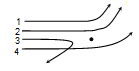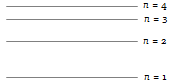The first line in the Lyman series has wavelength $\mathrm{\lambda }$. The wavelength of the first line in Balmer series is

(a) $\frac{2}{9}\mathrm{\lambda }$              (b) $\frac{9}{2}\mathrm{\lambda }$
(c) $\frac{5}{27}\mathrm{\lambda }$            (d) $\frac{27}{5}\mathrm{\lambda }$

Concept Questions :-

Spectral series
High Yielding Test Series + Question Bank - NEET 2020

Difficulty Level:

In the following transitions, which one has higher frequency

(a) 3 – 2              (b) 4 – 3
(c) 4 – 2              (d) 3 – 1

Concept Questions :-

Bohr's model of atom
High Yielding Test Series + Question Bank - NEET 2020

Difficulty Level:

The diagram shows the path of four $\alpha$-particles of the same energy being scattered by the nucleus of an atom simultaneously. Which of these are/is not physically possible?(a) 3 and 4            (b) 2 and 3
(c) 1 and 4            (d) 4 only

Concept Questions :-

Various atomic model
High Yielding Test Series + Question Bank - NEET 2020

Difficulty Level:

An electron jumps from 5th orbit to 4th orbit of hydrogen atom. Taking the Rydberg constant as  ${10}^{7}$ per metre. What will be the frequency of radiation emitted

(a)               (b)
(c)               (d) None of these

Concept Questions :-

Bohr's model of atom
High Yielding Test Series + Question Bank - NEET 2020

Difficulty Level:

Four lowest energy levels of H-atom are shown in the figure. The number of possible emission lines would be(a) 3             (b) 4
(c) 5             (d) 6

Concept Questions :-

Spectral series
High Yielding Test Series + Question Bank - NEET 2020

Difficulty Level:

The order of the size of nucleus and Bohr radius of an atom respectively are
(a)                (b)
(c)                (d)

Concept Questions :-

Bohr's model of atom
High Yielding Test Series + Question Bank - NEET 2020

Difficulty Level:

The ratio of the wavelengths for 2 $\to$ 1 transition in ${\mathrm{Li}}^{++}$${\mathrm{He}}^{+}$ and H is-
(a) 1 : 2 : 3            (b) 1 : 4 : 9
(c) 4 : 9 : 36           (d) 3 : 2 : 1

Concept Questions :-

Spectral series
High Yielding Test Series + Question Bank - NEET 2020

Difficulty Level:

The wavelength of light emitted from second orbit to first orbits in a hydrogen atom is
(a)          (b)
(c)           (d)

Concept Questions :-

Bohr's model of atom
High Yielding Test Series + Question Bank - NEET 2020

Difficulty Level:

Energy of the electron in nth orbit of hydrogen atom is given by ${\mathrm{E}}_{\mathrm{n}}=-\frac{13.6}{{\mathrm{n}}^{2}}\mathrm{eV}$. The amount of energy needed to transfer electron from first orbit to third orbit is

(a) 13.6 eV             (b) 3.4 eV
(c) 12.09 eV            (d) 1.51 eV

Concept Questions :-

Bohr's model of atom
High Yielding Test Series + Question Bank - NEET 2020

Difficulty Level:

The de-Broglie wavelength of an electron in the first Bohr orbit is
(a) Equal to one fourth the circumference of the first orbit
(b) Equal to half the circumference of the first orbit
(c) Equal to twice the circumference of the first orbit
(d) Equal to the circumference of the first orbit

Concept Questions :-

Bohr's model of atom